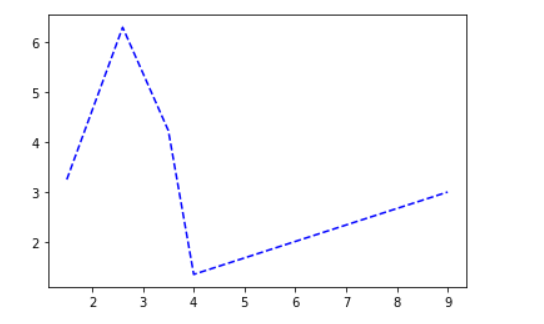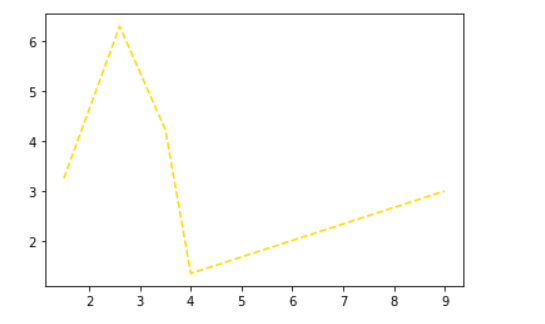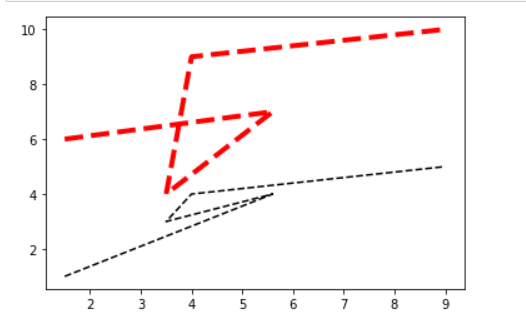# How to plot a dashed line in matplotlib?

• Last Updated : 31 Jan, 2022

Prerequisites: Matplotlib

In this article, we will see how to plot a dashed line in matplotlib. Matplotlib dashed line is a special styled line chart that represents the relationship between the X-axis and Y-axis with the help of linestyle dashed, we can also set a different color for each line and different linewidth. Let us understand it with the different examples below:

To plot dashed datapoint:

Syntax: matplotlib.pyplot.plot(x, y, linestyle=’dashed’)

• x:  X-axis points on the line.
• y:  Y-axis points on the line.
• linestyle: Change the style of the line.
• linewidhth: set width of a dash line.

### Example 1: Plotting a dashed line in matplotlib

To plot the dashed line we will create the dataset and then we use the above syntax to plot dashed datapoints.

Syntax: plt.plot(linestyle=’dashed’)

## Python3

 `# Import libraries``import` `matplotlib.pyplot as plt` `# Define Axes``x_points ``=` `[``1.5``, ``2.6``, ``3.5``, ``4``, ``9``]``y_points ``=` `[``3.25``, ``6.3``, ``4.23``, ``1.35``, ``3``]` `# Plot a graph``plt.plot(x_points, y_points, linestyle``=``'dashed'``)` `# Display graph``plt.show()`

Output:### Example 2: Customizing color dashed line in matplotlib

To customize we will use color attributes.

Syntax: plt.plot(linestyle=’–’, color=’gold’)

## Python3

 `# Import libraries``import` `matplotlib.pyplot as plt` `# Define Axes``X ``=` `[``1.5``, ``2.6``, ``3.5``, ``4``, ``9``]``Y ``=` `[``3.25``, ``6.3``, ``4.23``, ``1.35``, ``3``]` `# Plot a graph``plt.plot(X, Y, linestyle``=``'--'``, color``=``'gold'``)` `# Display graph``plt.show()`

Output:### Example 3: Customizing datapoints dashed line in matplotlib

linewidth attributes can be used to set width between datapoints.

Syntax: plt.plot(linestyle=’dashed’, linewidth= int)

## Python3

 `# Import libraries``import` `matplotlib.pyplot as plt` `# Define Axes``X ``=` `[``1.5``, ``5.6``, ``3.5``, ``4``, ``9``]``Y1 ``=` `[``1``, ``4``, ``3``, ``4``, ``5``]``Y2 ``=` `[``6``, ``7``, ``4``, ``9``, ``10``]` `# Plot a graph``plt.plot(X, Y1, linestyle``=``'dashed'``, color``=``'black'``)``plt.plot(X, Y2, linestyle``=``'dashed'``,``         ``color``=``'red'``, linewidth``=``4``)` `# Display graph``plt.show()`

OutputMy Personal Notes arrow_drop_up